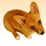# Masking NetCDF file with shapefile

3075
0
02-07-2013 01:48 PMNew Contributor III
Hello,

I am trying to create a tool which will accept a shapefile, and then use this shapefile as a mask for calculating the mean of various netcdf files.  I have read up on the gdal library, but I am very confused on how to use these tools.  Instead I have tried to do it with the tools available in arcgis.  What I have done in my python script is convert the input shapefile into a raster where all values are 1, then I use the arcpy.RasterToNumpyArray command to convert the raster to a numpy array. I then multiply this array by the arrays I have created from the netcdf files.  So the product of the numpy array of the shapefile and the numpy array of the netcdf file should be the array of the netcdf file within the polygon extent, because I masked out all values outside the polygon extent as not a number. The tool runs successfully but my results are off.  I have noticed that when I convert the raster of the polygon to a numpy array, the numpy array is much larger than the raster and original polygon.  Does anyone know why the RasterToNumpyArray tool would create an array that is much bigger than the raster?  The reason I know it is much bigger is because when I convert the product of the numpy arrays back to a raster using the NumpyArrayToRaster command, it is the shape of my input shapfile, but just much larger, and with the same lower left hand corner point.  I would really appreciate and insight on what I might be doing wrong.  Thank you!
Tags (3)
0 Replies